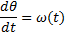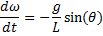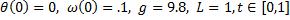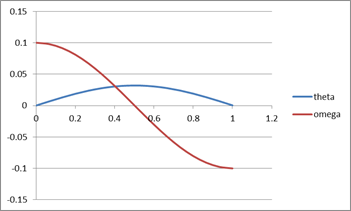# =ODEVAL(col1, exprn, opr, pt, [options])

• Use ODEVAL to compute a single value from a solution array obtained by any of the ordinary differential equations solvers. ODEVAL computes the value using a specified calculus operation including interpolation, differentiation or integration.

• In a dynamical optimization problem, ODEVAL can be used to define dynamic constraints on the solution array of the ordinary differential equations solver. A constraint formula is typically defined as the difference between the computed value by ODEVAL and a target value. The constraint formulas are input to NLSOLVE.

### Required Inputs

col1 a reference to the first column in the solution array. This defines the time or spatial range for the calculus operation.

You may select a sub range of column 1 although this reduces the accuracy of the calculus operation.

exprn a reference to the operand for the calculus operation.

The reference may be to single variable or a formula implicating mulutiple variables. If your operand is a single variable, just pass its name in this argument.

opr a string value specifying the calculus operation to perform. Supported values are INTERP, DERIV, and INTEG.

pt defines the point of interpolation or differentiation for the calculus operation.

Not required if the calculus operation is INTEG.

### Optional Inputs

order controls the calculus operation as described below:

• If opr is INTERP or INTEG
Enter 1 to use linear splines, or 3 to use cubic splines. Default is 3.

• If opr is DERIV
Enter the derivative order: 1, 2 or 3. Default is 1.

Consider the array result shown in Table 1 from the simulation of an initial value pendulum problem with IVSOLVEWith the following parameters:A B C 6 T1 theta omega 7 0 0 0.1 8 0.066667 0.006619 0.097835 9 0.133333 0.012949 0.091411 10 0.2 0.018719 0.081034 11 0.266667 0.023675 0.067133 12 0.333333 0.027606 0.050324 13 0.4 0.030336 0.031328 14 0.466667 0.031752 0.010976 15 0.533333 0.031789 -0.00985 16 0.6 0.030448 -0.03025 17 0.666667 0.027784 -0.04934 18 0.733333 0.023917 -0.06629 19 0.8 0.01901 -0.08036 20 0.866667 0.013279 -0.09095 21 0.933333 0.006971 -0.09759 22 1 0.000361 -0.09999You can use ODEVAL() to extract or compute values of interest from the simulation result:

 Objective Formula Result Find derivative of omega at t = 0.5 =ODEVAL(A7:A22,omega,"deriv",0.5) -0.313005332 Integrate theta from 0 to 1 =ODEVAL(A7:A22,theta,"integ") 0.020409729 Interpolate theta at t = 0.5 =ODEVAL(A7:A22,theta,"interp",0.5) 0.031944316 Integrate the square of omega from 0 to 1 =ODEVAL(A7:A22,H21,"integ") 0.004982081 H21 =omega^2

When solving a parametrized dynamical optimization problem, ODEVAL is used to define constraints on the dynamical system solution typically as the difference between a computed value by ODEVAL and a target value. You can then use the nonlinear solver NLSOLVE to find optimal values for the design parameters that will minimize the differences your defined constraints.

Question or Comment? Email us:
support @ excel-works.comExceLab: Transforming Excel into a Calculus Power House
ExceLab functions and methods are protected by USA Patents 9286286, 9892108, 10114812 and pending.